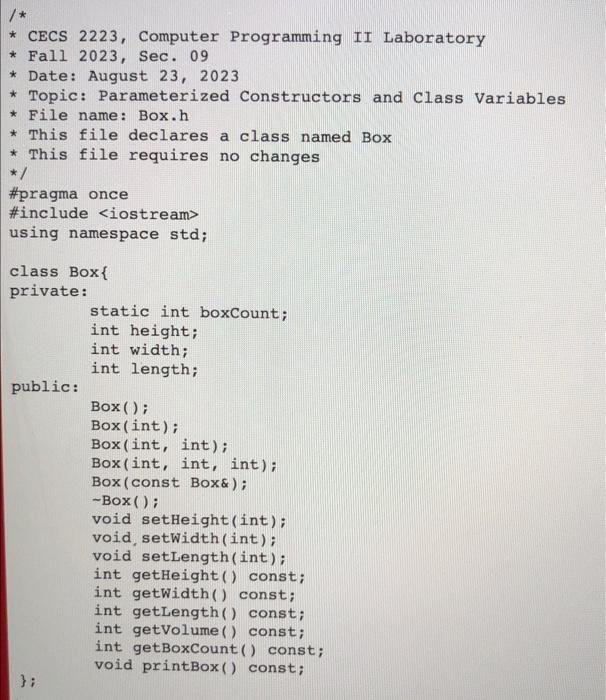Rating : ⭐⭐⭐⭐⭐
Price : \$10.99
Language:EN
Pages: 7
Words: 1227

# Complete "Box" Class Code Homework Answers Needed# Step By Step Answers with Explanation

#pragma once

#include <iostream>

int height;

int width;

Box(int, int); // 2-parameter constructor

Box(int, int, int); // 3-parameter constructor

void setLength(int);

int getHeight() const;

void printBox() const;

};

boxcount++;

}

boxcount++;

}

if (width < 0) {

width = 0;

if (height < 0) {

height = -1;

if (length < 0) {

length = 0;

boxcount++;

}

height = (h >= 0) ? h : height;

}

length = (l >= 0) ? l : length;

}

return width;

}

return height * width * length;

}

cout << "Height: " << height << "\tWidth: " << width << "\tLength: " << length << "\tVolume: " << getVolume() << endl;

}

box1.setHeight(1);

box1.setWidth(10);

Box box3(3, 4); // 2-parameter constructor

box3.setLength(12);

cout << "HEIGHT\tWIDTH\tLENGTH\tVOLUME" << endl;

box1.printBox();

cout << "Program developed by [YOUR NAME], ID: [YOUR ID NUMBER]" << endl;

return 0;

How It Works